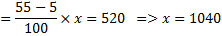## Percentage For SBI PO : Set – 18

1) 65% of a number is more than its 2/5 th by 140. What is 30% of that number?

a) 186

b) 168

c) 164

d) 182

e) None of these

Click Here To View Answer

b)

According to question,

0.65x= 0.4x+140

0.25x=140-> x=560

Required answer =560*30/100=168

2) If the numerator of a fraction  is increased by 350% and the denominator of the fraction is increased by 300%, the resultant fraction is 9/22 . What is the original fraction?

a) 3/4

b) 5/12

c) 7/9

d) 4/11

e) None of these

Click Here To View Answer

d)

According to question,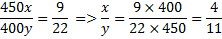3) In an examination Nisha scores a total of 555 marks out of 850. What is her approximate percentage in the examination?

a) 59

b) 72

c) 68

d) 65

e) 70

Click Here To View Answer

d)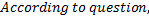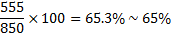4) 64% of a number is 2592. What is 88% of that number?

a) 3458

b) 3202

c) 3826

d) 3564

e) None of these

Click Here To View Answer

d)

According question,

0.64x=2592-> x=4050

Required answer,

4050*88/100=3564

5) Population of a village in year 2007 was 180000. In 2008 it became 210000. What was the percent rise in population from 2007 to 2008?

a) 15 1/3

b) 14.29

c) 16 2/3

d) 16.25

e) None of these

Click Here To View Answer

C)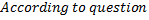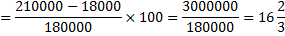6) Five-ninth of sixty two percent of a number is equal to 2790. What is the number?

a) 8100

b) 7200

c) 6300

d) 8500

e) None of these

Click Here To View Answer

a)

According question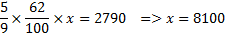7) What is the value of 72% of two-fifth of 450?

a) 648.4

b) 129.6

c) 324.2

d) 162.6

e) None of these

Click Here To View Answer

b)

According to question,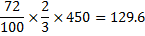8) What is seventy five percent of three-fourth of 1600?

a) 1000

b) 500

c) 450

d) 900

e) None of these

Click Here To View Answer

d)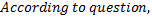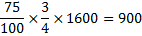9) Disha scored 35 marks in Geography, 32 marks in History, 29 marks in Science, 40 marks in Mathematics, 36 marks in English and 33 marks in Hindi. The maximum marks in each subject are 50. What is Disha’s approximate percentage

a) 68

b) 63

c) 58

d) 53

e) 76

Click Here To View Answer

a)

According to question,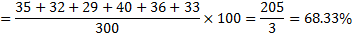10) In an examination it is required to get 55% of the aggregate marks to pass. A student gets 520 marks and is declared failed by 5% marks. What are the maximum aggregate marks a student can get?

a) 960

b) 1250

c) 1040

d) Cannot be determined

e) None of these

Click Here To View Answer

C)

According to question,